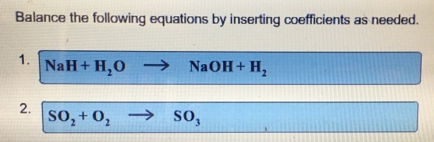# Problem: Balance the following equations by inserting coefficients as needed. 1. NaH + H2O → NaOH + H22. SO2 + O2 → SO3

🤓 Based on our data, we think this question is relevant for Professor Saleh's class at NVCC.

###### FREE Expert Solution

NaH + H2O → NaOH + H(Already balanced)###### Problem Details

Balance the following equations by inserting coefficients as needed.

1. NaH + H2O → NaOH + H2

2. SO2 + O2 → SO3